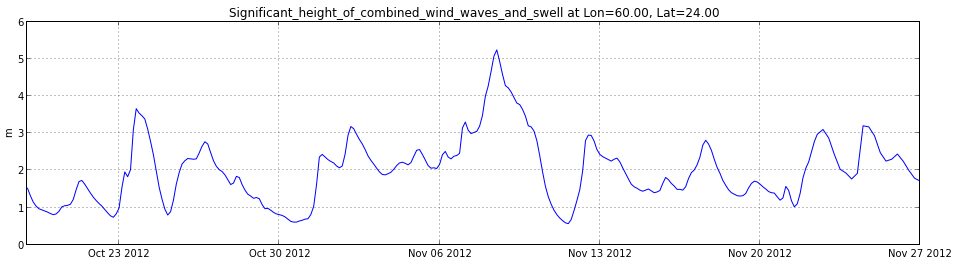## Extract time series from 3D [time,lat,lon] dataset¶

In [ ]:
import numpy as np
import matplotlib.pyplot as plt
import netCDF4

In [ ]:
# Global NCEP WaveWatch 3 dataset from Unidata's THREDDS Collection:
# http://motherlode.ucar.edu:8080/thredds/catalog.html
url='http://motherlode.ucar.edu:8080/thredds/dodsC/fmrc/NCEP/WW3/Global/NCEP-WW3-Global_best.ncd'

In [ ]:
# desired station time series location
loni = 60.00833
lati = 24.00833

In [ ]:
nc = netCDF4.Dataset(url)
nc.variables

In [ ]:
lat = nc.variables['lat'][:]
lon = nc.variables['lon'][:]
times = nc.variables['time']
jd = netCDF4.num2date(times[:],times.units)

In [ ]:
# function to find index to nearest point
def near(array,value):
idx=(np.abs(array-value)).argmin()
return idx

In [ ]:
# find nearest point to desired location
ix = near(lon, loni)
iy = near(lat, lati)

In [ ]:
# get all time records of variable [vname] at indices [iy,ix]
vname = 'Significant_height_of_combined_wind_waves_and_swell'
var = nc.variables[vname]
h = var[:,iy,ix]

In :
plt.figure(figsize=(16,4))
plt.plot_date(jd,h,fmt='-')
plt.grid()
plt.ylabel(var.units)
plt.title('%s at Lon=%.2f, Lat=%.2f' % (vname, lon[ix], lat[iy]))

Out:
<matplotlib.text.Text at 0x7f25f5a3e5d0>